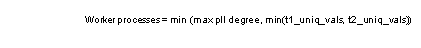Worker processes for range-based scans

A merge join can use multiple worker processes to perform:

• The scan that selects rows into a worktable, for any merge join that requires a sort

• The worktable sort

• The merge join and subsequent joins in the step

• The range scan of both tables during a full merge join

Usage while creating the worktable

If a worktable is needed for a merge join, the query step that creates the worktable can use a serial or parallel access method for the scan. The number of worker processes for this step is determined by the usual methods for selecting the number of worker processes for a query. The query that selects the rows into the worktable can be a single-table query or a join performing a nested-loop or merge join, or a combination of nested-loops joins and a merge join.

Parallel sorting for merge-join worktables

Parallel sorting is used when the number of pages in the worktable to be sorted is eight times the value of the number of sort buffers configuration parameter.

For the merge step, the number of merge threads is set to max parallel degree, unless the number of distinct values is smaller than max parallel degree. If the number of values to be merged is smaller than the max parallel degree, the task uses one worker process per value, with each worker process merging one value. If the tables being merged have different numbers of distinct values, the lower number determines the number of worker processes to be used. The formula is:When there is only one distinct value on the join column, or there is an equality search argument on a join column, the merge step is performed in serial mode. If a merge join is used for this query, the merge is performed in serial mode:

select * from t1, t2
where t1.c1 = t2.c1
and t1.c1 = 10

Total usage for merge joins

A merge join can use up to max parallel degree threads for the merge step and up to max parallel degree threads can be used for each sort. A merge that performs a parallel sort may use up to 2*max parallel degree threads. Worker processes used for sorts are released when the sort completes.